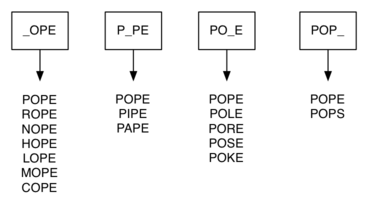# 7.8. Building the Word Ladder Graph¶

Our first problem is to figure out how to turn a large collection of words into a graph. What we would like is to have an edge from one word to another if the two words are only different by a single letter. If we can create such a graph, then any path from one word to another is a solution to the word ladder puzzle. Figure 1 shows a small graph of some words that solve the FOOL to SAGE word ladder problem. Notice that the graph is an undirected graph and that the edges are unweighted.

We could use several different approaches to create the graph we need to solve this problem. Let’s start with the assumption that we have a list of words that are all the same length. As a starting point, we can create a vertex in the graph for every word in the list. To figure out how to connect the words, we could compare each word in the list with every other. When we compare we are looking to see how many letters are different. If the two words in question are different by only one letter, we can create an edge between them in the graph. For a small set of words that approach would work fine; however, let’s suppose we have a list of 5,110 words. Roughly speaking, comparing one word to every other word on the list is an $$O(n^2)$$ algorithm. For 5,110 words, $$n^2$$ is more than 26 million comparisons.

We can do much better by using the approach shown in Figure 2. Suppose that we have a number of buckets, each labeled with a four-letter word, except that one of the letters on the label has been replaced by an underscore. As we process a list of words, we compare each word with each bucket using the underscore (_) as a wildcard. Every time we find a matching bucket we put the word in that bucket, so that both POPE and POPS would both go into the POP_ bucket. Once we have all the words in the appropriate buckets, we know that all the words in each bucket must be connected.Figure 2: Word Buckets for Words That Differ by One Letter¶

In Python, we can implement the scheme we have just described by using a dictionary. The labels on the buckets we have just described are the keys in our dictionary. The value stored for each key is a list of words. Once we have the dictionary built, we can create the graph. We start our graph by creating a vertex for each word in the graph. Then we create edges between all the vertices we find for words found under the same key in the dictionary. Listing 1 shows the Python code required to build the graph.

Listing 1

from pythonds3.graphs import Graph

def build_graph(filename):
buckets = {}
the_graph = Graph()
with open(filename, "r", encoding="utf8") as file_in:
# create buckets of words that differ by 1 letter
for line in all_words:
word = line.strip()
for i, _ in enumerate(word):
bucket = f"{word[:i]}_{word[i + 1 :]}"

Since this is our first real-world graph problem, you might be wondering how sparse the graph is. The list of four-letter words we have for this problem is 5,110 words long. If we were to use an adjacency matrix, the matrix would have $$5,110 \cdot 5,110$$ = 26,112,100 cells. The graph constructed by the build_graph function has exactly 53,286 edges, so the matrix would have only 0.20% of the cells filled! That is a very sparse matrix indeed.